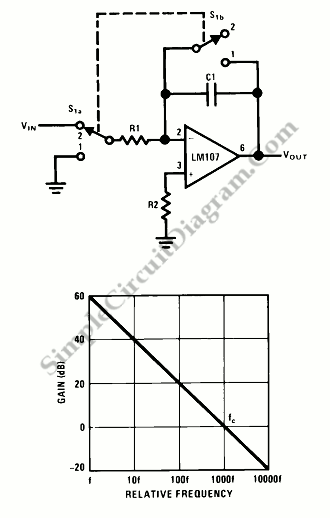# Op-Amp Circuit: Integrator

This is a Op-Amp Integrator Circuit. This circuit is used to perform the integration operation. Essentially, this circuit is a low-pass filter with a frequency response decreasing at 6 dB per octave. This circuit should be equipped with an external establishing initial conditions’s method (s1). Here is the circuit:When S1 is in position 2, the amplifier is connected as an integrator. So the output will change in accordance with a constant times the time integral of the input voltage. When S1 is in position 1, the amplifier is connected in unity-gain and discharges C1, initial condition is set to zero volts.

Following equations show the output voltage and frequency cut off:
Vout=(1/(R1C1))*integral(Vin,dt,t1,t2)
Fc=1/(2piR1C1)
R1=R2

There are two cautions those should be observed, they are R2 must equal R1 for minimum error due to bias current and the amplifier used should generally be stabilized for unity-gain operation. [Source: National Semiconductor Application Note]